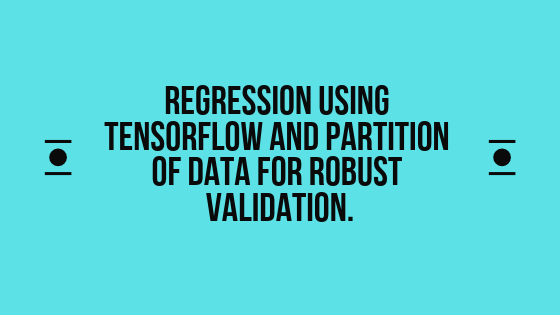# Regression using Tensorflow and partition of data for robust validation.

2379Again in the tutorial will use Gradient descent optimization algorithm. Additionally, we will divide our data set into three slices, Training, Testing, and validation. In our example, we have data in CSV format with columns “height weight age projects salary”. Assuming there is a correlation between projects and salary will try to predict the salary given projects completed. You download data using this link: “https://drive.google.com/file/d/1Gx0riTlJHt9o_VyokrKNbj384AhwXpAW/view?usp=sharing”

## Importing essential libraries

``````from __future__ import print_function

import math ##For basic mathematical operations

from IPython import display ## Plot setup for Ipython
from matplotlib import cm ##  Colormap reference
from matplotlib import gridspec ##plot setups
from matplotlib import pyplot as plt ##plot setups
import numpy as np
import pandas as pd
from sklearn import metrics
import tensorflow as tf
from tensorflow.python.data import Dataset

drive.mount('/content/gdrive') ## Mounting drive

tf.logging.set_verbosity(tf.logging.ERROR)
pd.options.display.max_rows = 10
pd.options.display.float_format = '{:.1f}'.format``````

``````dataframe = pd.read_csv("/content/gdrive/My Drive/Colab Notebooks/TENSOR_FLOW/train_dataset.csv", sep=",")
#dataframe["height"] = dataframe["height"]*-1
dataframe = dataframe.reindex(
np.random.permutation(dataframe.index))
`height    weight  age projects    salary 1623    117.2   33.1    7   2015    279600 12851    121.8   37.4    37  1569    286200 10236    119.9   38.9    24  235 136800 2783    117.7   34.1    29  1216    134300 16170    122.5   37.8    40  1675    330000`

## Step 2: Preprocess features

This step is optional and can be used to create synthetic features we will cover this in upcoming posts.

``````def preprocess_features(dataframe):

selected_features = dataframe[
["height",
"weight",
"age",
"projects"]]

processed_features = selected_features.copy()
# Create a synthetic feature.
processed_features["height_weight"] = (
dataframe["height"] /
dataframe["weight"])
return processed_features

def preprocess_targets(dataframe):

output_targets = pd.DataFrame()
# Scale the target to be in units of thousands of dollars.
output_targets["salary"] = (
dataframe["salary"] / 1000.0)
return output_targets``````

## Step 3: Split data-set

Choose the first 12000 (out of 17000) examples for training then choose the last 5000 (out of 17000) examples for validation.

``````training_examples = preprocess_features(dataframe.head(12000))

validation_examples = preprocess_features(dataframe.tail(5000))
validation_targets = preprocess_targets(dataframe.tail(5000))

print("Training examples summary:")
display.display(training_examples.describe())
print("Validation examples summary:")
display.display(validation_examples.describe())

print("Training targets summary:")
display.display(training_targets.describe())
print("Validation targets summary:")
display.display(validation_targets.describe())``````
`Training examples summary: height    weight  age projects    height_weight count    12000.0 12000.0 12000.0 12000.0 12000.0 mean    119.6   35.6    28.7    1423.6  3.4 std    2.0 2.1 12.6    1168.4  0.1 min    114.3   32.5    1.0 3.0 2.9 25%    118.0   33.9    18.0    788.0   3.2 50%    118.5   34.2    29.0    1160.0  3.5 75%    121.8   37.7    37.0    1704.0  3.5 max    124.3   41.9    52.0    35682.0 3.6 Validation examples summary: height    weight  age projects    height_weight count    5000.0  5000.0  5000.0  5000.0  5000.0 mean    119.6   35.6    28.3    1443.8  3.4 std    2.0 2.1 12.6    1096.9  0.1 min    114.6   32.5    2.0 6.0 2.9 25%    118.0   33.9    18.0    792.0   3.2 50%    118.5   34.3    28.0    1185.0  3.4 75%    121.8   37.7    37.0    1756.0  3.5 max    124.3   42.0    52.0    11956.0 3.6 Training targets summary: salary count    12000.0 mean    207.6 std    115.9 min    15.0 25%    119.8 50%    180.5 75%    266.2 max    500.0 Validation targets summary: salary count    5000.0 mean    206.5 std    116.1 min    15.0 25%    119.1 50%    179.9 75%    262.5 max    500.0`

## Step 4: Feature construction

``````def construct_feature_columns(input_features):
return set([tf.feature_column.numeric_column(my_feature)
for my_feature in input_features])

def my_input_fn(features, targets, batch_size=1, shuffle=True, num_epochs=None):
features = {key:np.array(value) for key,value in dict(features).items()}

ds = Dataset.from_tensor_slices((features,targets)) # warning: 2GB limit
ds = ds.batch(batch_size).repeat(num_epochs)

if shuffle:
ds = ds.shuffle(10000)

features, labels = ds.make_one_shot_iterator().get_next()
return features, labels``````

## Step 5: Training model

``````def train_model(
learning_rate,
steps,
batch_size,
training_examples,
training_targets,
validation_examples,
validation_targets):

periods = 10
steps_per_period = steps / periods

# linear regressor object.
linear_regressor = tf.estimator.LinearRegressor(
feature_columns=construct_feature_columns(training_examples),
optimizer=my_optimizer
)

# input functions.
training_input_fn = lambda: my_input_fn(training_examples,
training_targets["salary"],
batch_size=batch_size)
predict_training_input_fn = lambda: my_input_fn(training_examples,
training_targets["salary"],
num_epochs=1,
shuffle=False)
predict_validation_input_fn = lambda: my_input_fn(validation_examples,
validation_targets["salary"],
num_epochs=1,
shuffle=False)

# Train the model
print("Training model...")
print("RMSE (on training data):")
training_rmse = []
validation_rmse = []
for period in range (0, periods):
# Train the model, starting from the prior state.
linear_regressor.train(
input_fn=training_input_fn,
steps=steps_per_period,
)

training_predictions = linear_regressor.predict(input_fn=predict_training_input_fn)
training_predictions = np.array([item['predictions'] for item in training_predictions])

validation_predictions = linear_regressor.predict(input_fn=predict_validation_input_fn)
validation_predictions = np.array([item['predictions'] for item in validation_predictions])

.
training_root_mean_squared_error = math.sqrt(
metrics.mean_squared_error(training_predictions, training_targets))
validation_root_mean_squared_error = math.sqrt(
metrics.mean_squared_error(validation_predictions, validation_targets))

print("  period %02d : %0.2f" % (period, training_root_mean_squared_error))

training_rmse.append(training_root_mean_squared_error)
validation_rmse.append(validation_root_mean_squared_error)
print("Model training finished.")

# Plots.
plt.ylabel("RMSE")
plt.xlabel("Periods")
plt.title("Root Mean Squared Error vs. Periods")
plt.tight_layout()
plt.plot(training_rmse, label="training")
plt.plot(validation_rmse, label="validation")
plt.legend()

return linear_regressor``````

### Supply feature and train model

``````minimal_features = ["height_weight","projects"]

assert minimal_features, "You must select at least one feature!"

minimal_training_examples = training_examples[minimal_features]
minimal_validation_examples = validation_examples[minimal_features]

#
# Don't forget to adjust these parameters.
#
train_model(
learning_rate=0.001,
steps=500,
batch_size=5,
training_examples=minimal_training_examples,
training_targets=training_targets,
validation_examples=minimal_validation_examples,
validation_targets=validation_targets)``````
`Training model… RMSE (on training data):   period 00 : 188.03   period 01 : 203.27   period 02 : 203.27   period 03 : 188.01   period 04 : 187.99   period 05 : 194.65   period 06 : 194.66   period 07 : 183.70   period 08 : 183.70   period 09 : 196.58 Model training finished.`

1.2.Hi Nilesh, Nice work with the computer generated Bible. I had a similar thought with another piece of art. Wanted…

3.4.5.I am Nilesh Kumar, a graduate student at the Department of Biology, UAB under the mentorship of Dr. Shahid Mukhtar. I joined UAB in Spring 2018 and working on Network Biology. My research interests are Network modeling, Mathematical modeling, Game theory, Artificial Intelligence and their application in Systems Biology. I graduated with master’s degree “Master of Technology, Information Technology (Specialization in Bioinformatics)” in 2015 from Indian Institute of Information Technology Allahabad, India with GATE scholarship. My Master’s thesis was entitled “Mirtron Prediction through machine learning approach”. I worked as a research fellow at The International Centre for Genetic Engineering and Biotechnology, New Delhi for two years.